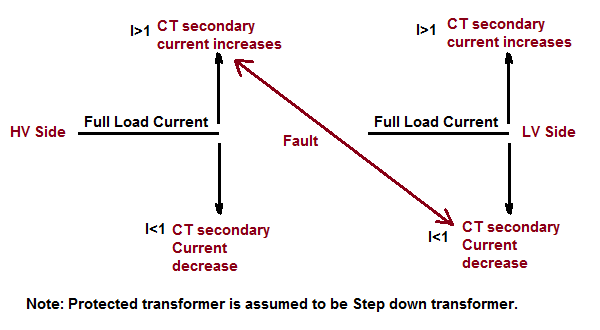# CT Matching Factor in Transformer Differential Protection

CT Matching Factor is basically a multiplication factor by which secondary current of CTs on HV side and LV side is multiplied to make it equal to rated secondary current. This means, if the rated CT secondary current is 1A, then multiplication of CT secondary current by matching factor will give unity. To get this multiplication factor, a reference current is assumed for HV and LV side separately. This reference current is Full Load current.

Before going into the detail of CT matching factor, let us first discuss its requirement. As we know that in differential protection, phasor sum of CT secondary current are considered for calculation of differential current. In transformer differential protection, the CT ratio in HV and LV side are different, therefore merely taking the phasor sum will result in some definite differential current which may be more than the low set differential setting and hence will lead to actuation of differential element of relay even under normal operating condition. Therefore, it is very important to match the CT secondary current so that differential current is zero under normal operating condition. Let us understand this with an example.

Let a 70 MVA, 220 / 6.9 kV transformer is to be protected by differential protection. Let us assume that the CT ratio for HV side and LV side are 400/1 and 3200/1 respectively.

HV side full load current of transformer

= (70×103) / (220×1.732)

= 183.7 A

CT secondary current corresponding to HV side full load current

= 183.7 / 400

= 0.46 A

LV side full load current of transformer

= (70×103) / (6.9×1.732)

= 5857 A

CT secondary current corresponding to LV side full load current

= 5857 / 3200

= 1.83 A

Thus the differential current under the considered full load condition

= (1.83 – 0.46)

= 1.37 A

Normally the differential setting for transformer is 0.2 pu, this means the differential element will operate. To avoid such an occurrence it is important to match the HV and LV side CT secondary current so that their phasor sum may be zero under normal situation. Also, the rated CT secondary current is 1 A, we need to limit the CT secondary current to 1. Multiplication of actual CT secondary current by matching factor under various normal operating conditions will limit it to 1.

So what will we do to match? We will introduce a term called Matching Factor (MF). What this Matching Factor will do is that when we multiply the HV and LV side CT secondary current corresponding to full load current by this matching factor, the result will be 1. Let us now calculate this.

As CT secondary current corresponding to full load current in HV side is 0.46 A, therefore

0.46xMF = 1

MF = 1/0.46

= 2.17

Similarly, the matching factor for LV side

MF = 1/1.83

= 0.546

As we take full load current for calculating the matching factor, therefore its general formula may be obtained as

Full Load Current x MF = Rated CT Secondary Current

Considering rated CT secondary current equal to 1,

MF = 1 / Full Load Current

For differential current calculation, relay uses this matching factor to get the current in HV and LV side. This means if the differential current as calculated in relay is 0.2 pu then the actual HV side primary current will be

(0.2 / MF)x400 A

= (0.2 / 2.17)x400 A

= 36.86 A

You might be wondering that this is the single phase pick-up value of differential element. Yes, this is. See, how we have arrived at the value of single phase pick-up. The formula for single phase pick-up may thus be written as

Single phase Pick-up Value in terms of CT secondary

= Idiff / MF

### Significance of CT Matching Factor:

Let us consider a fault condition within the protected zone. If transformer is working as step down transformer, the fault current will be much higher than the full load current in HV side but lower than full load current in LV side.Calculated CT secondary current in HV side will be more than 1 and that in LV side will be less than 1. Thus under fault condition, the value of differential current will rise and subsequently it will actuate to isolate the transformer from the system.

### 10 thoughts on “CT Matching Factor in Transformer Differential Protection”

1.Yassin says:

Excellent Explanation
Thanks

1.admin says:

Thank you Yassin. Please share if you like it.

2.Aaron Lal says:

Thanks for posting! Excellent explanation about the CT matching factor. Keep up the good work!

3.Manu Das says:

well explained. very easy to understand. nice post.

4.Sana Vinod Kumar says:

sir,
you explanation was good but in the article CT secondary current corresponding to LV side full load current

= 5857 / 400

= 1.83 A

but actually there you have to type =5857/3200 =>1.83

1.admin says:

5.Logu says:

hi sir,
calculation is very useful, but u can explain in different CT sy ratio adopted.
HV secondary ct is 1A and LV secondary ct is 5A.

1.admin says:

Example is just taken to illustrate the idea.

6.Sh. S says:

How can I calculate ct matching for duff protection

1.Sh. S says:

CT matching for duff protection

This site uses Akismet to reduce spam. Learn how your comment data is processed.